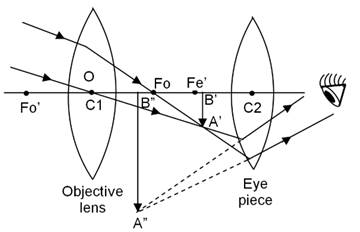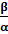### Explain the working of an astronomical telescope using refraction of light.

Question 5.

Explain the working of an astronomical telescope using refraction of light.

Answer:

In the astronomical telescopes the image is formed by bending of light also known as refraction so these telescopes are known as refracting telescopes. It is used to see the magnified images of heavenly bodies like stars, planets, etc.

The construction of the telescope is given as:

An astronomical telescope is made up of two convex lenses :

i) an objective lens O and,

ii) an eye piece E.

The focal length fo of the objective lens of astronomical telescope is large as compared to the focal length fe of the eye piece.

Working of the telescope:

The ray diagram to show the working of the astronomical telescope is shown in figure below:i. A parallel beam of light from a star or a satellite falls on the objective lens of the telescope.

ii. The objective lens forms a real, inverted and diminished image A’B’ of the heavenly body.

iii. This image (A’B’) now acts as an object for the eye piece E, whose position is adjusted so that the image lies between the focus fe’ and the optical centre C2 of the eye piece.

iv. Now the eye piece forms a virtual, inverted and highly magnified image of object at infinity. When the final image of an object is formed at infinity, the telescope is said to be in ‘normal adjustment’.

It should be noted that, the final image of object (such as stars, planets or satellites) formed by an astronomical telescope is always inverted with respect to the object. But it does not matter whether the image formed by an astronomical telescope is inverted or not, as all the heavenly bodies are usually spherical is shape. Astronomical telescopes bend light called as refraction. Due to this refraction the light rays which are parallel, converge at a focal point and those lines that are not parallel converge upon focal plane. The telescope converts a bundle of parallel rays and makes angle α and angle β with a second parallel bundle. The ratio ofgives angular magnification to the telescope.